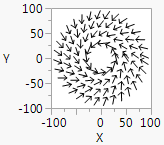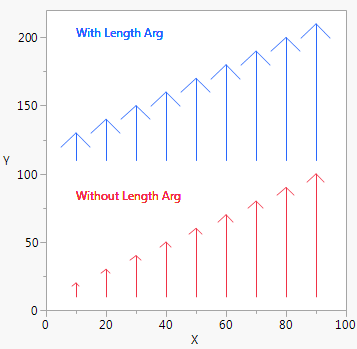Publication date: 05/24/2021

## Draw Arrows

Arrow() draws an arrow from the first point to the second point. The default arrowhead is scaled to 1 plus the square root of the length of the arrow. To set the length of the arrowhead, add an optional first argument, specifying the length of the arrowhead in pixels. The following example draws simple arrows.

`win = New Window( "Arrowheads",`
`	Graph Box(`
`		Pen Size( 4 );`
` `

/* matrix 1 defines the x coordinates of an arrow

matrix 2 defines the y coordinates of an arrow */

`		Arrow( 20, [10 30 90], [88 22 44] );`
`	)`
`);`

The following example draws arrows in a circular pattern.

`win = New Window( "Hurricane",`
`	Graph Box(`
`		Frame Size( 100, 100 ),`
`		X Scale( -100, 100 ),`
`		Y Scale( -100, 100 ),`
`		For( r = 35, r < 100, r += 20,`
`			ainc = 2 * Pi() * 3 / r;`
`			For( a = 0, a < 2 * Pi(), a += ainc,`
`				x = r * Cosine( a );`
`				y = r * Sine( a );`
`				aa = a + ainc * 45 / r;`
`				rr = r - r / 6;`
`				x2 = rr * Cosine( aa );`
`				y2 = rr * Sine( aa );`
` `

/* list 1 defines the start point for the arrow

list 2 defines the end point for the arrow */

`				Arrow( {x, y}, {x2, y2} );`
`			);`
`		)`
`	)`
`);`

Figure 12.11 Drawing ArrowsThe following example compares drawing with a specified length (19 pixels) and drawing with the default arrow head size.

`win = New Window( "Arrowheads",`
`	Graph Box(`
`		Frame Size( 300, 300 ),`
`		X Scale( 0, 100 ),`
`		Y Scale( 0, 220 ),`
`		x = 10;`
`		y1 = 10;`
`		y2 = y1 + 10;`
`		For( i = 1, i < 10, i++,`
`			Pen Color( "Red" );`
`			Arrow( {x, y1}, {x, y2} );`
` `
`			y2 += 10;`
`			y1 += 100;`
`			y2 += 100;`
`			Pen Color( "Blue" );`
`			Arrow( 20, {x, y1}, {x, y2} );`
` `
`			x += 10;`
`			y1 -= 100;`
`			y2 -= 100;`
` `
`			Text Color( "Red" );`
`			Text( {10, 80}, "Without Length Arg" );`
` `
`			Text Color( "Blue" );`
`			Text( {10, 200}, "With Length Arg" );`
`		);`
`	)`
`);`